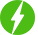# Solution of Simultaneous Equations

1

Solution of Simultaneous Equations, Simultaneous Equation.

## Course Description

Simultaneous linear equations occur quite often in engineering and science. Linear systems of equations are associated with many problems in engineering and science, as well as with applications of mathematics to the social sciences and quantitative study of business and economic problems.The analysis of electronic circuits consisting of invariant elements, analysis of a network under sinusoidal steady-state conditions, determination of the output of a chemical plant, and finding the cost of chemical reactions are some of the Exercises which depend on the solution of simultaneous linear algebraic equations. The solution of such equations can be obtained by Direct or Iterative methods. Linear algebraic equations occur in almost all branches of engineering. Their most important application

in engineering is in the analysis of linear systems (any system whose response is proportional to the input is deemed to be linear). Linear systems include structures, elastic solids, heat flow, seepage of fluids, electromagnetic fields and electric circuits i.e., most topics taught in an engineering curriculum. If the system is discrete, such as a truss or an electric circuit, then its analysis leads directly to linear algebraic equations.

In this course we will discuss the some direct and iterative methods to get the solutions.

Gauss elimination method, Thomas method, Jacobi’s iteration method and Gauss-Seidal iteration method.

Also you will be able to use calculator to get the solution in few seconds.

We will be happy to hear your thoughtsRegister New Account
• Total (0)
0